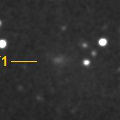# \$B1'ET5\WB@1(B

C/1997 T1 ( Utsunomiya )###\$B%W%m%U%#!<%k(B

 \$BH/8+F|(B 1997\$BG/(B10\$B7n(B3\$BF|(B \$BH/8+8wEY(B 10.5\$BEy(B \$BH/8+ \$B1'ET5\>O8c(B (\$B7'K\8)(B)

###\$B###\$B50F;MWAG(B

```   The following improved orbital elements by Kenji Muraoka, are
from 571 observations 1997 Oct. 5 to 1998 June 18, perturbations
by 9 Planets, Moon and 5 minor planets were taken into account.
The mean residual is +/- 0.69 arc seconds.

Epoch  =  1997 Dec. 18.0  TT       JDT = 2450800.5
T  =  1997 Dec. 10.14495       +/- 0.00004 (m.e.) TT
Peri. =   95.96559                +/- 0.00002
Node  =   53.70318                +/- 0.00002   (2000.0)
Incl. =  127.99265                +/- 0.00002
q  =    1.3591073              +/- 0.0000003 AU
e  =    0.9985255              +/- 0.0000016
1/a  =   +0.0010849              +/- 0.0000012 1/AU
( P  =  27984 years )
orig. 1/a  =   +0.001160   1/AU
fut.  1/a  =   +0.001313   1/AU
```

###\$B@1?^(B1997\$BG/(B 9\$B7n(B 9\$BF|!A(B1997\$BG/(B10\$B7n(B29\$BF|(B1997\$BG/(B10\$B7n(B29\$BF|!A(B1999\$BG/(B 1\$B7n(B22\$BF|(B

###\$B8wEYJQ2=(B

```        m1 = 6.5 + 5 log\$B&\$(B + 16.5 log r
```##### \$B50F;MWAG\$OB<2,7r<#;a\$N7W;;\$K\$h\$k\$b\$N\$G\$9!#(B \$B@1?^\$O(B StellaNavigator Ver.2.0 for Windows (\$B%"%9%H%m%"!<%D(B \$BJTCx(B / \$B%"%9%-!<=PHG6I4)(B) \$B\$G:n@.\$7\$?\$b\$N\$G\$9!#(B \$B8wEY%0%i%U\$O(BComet for Windows\$B\$G:n@.\$7\$?\$b\$N\$G\$9!#(B